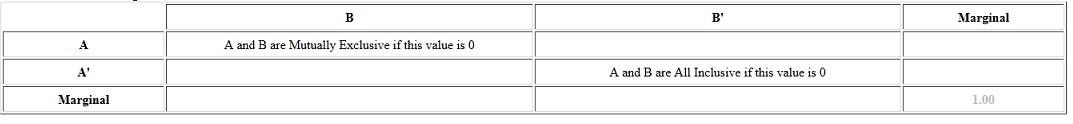#### Concept of Addition and Multiplication Rules

Probability Rules:

‘OR’ or Unions:

Mutually Exclusive Events:

Two events are mutually exclusive when they cannot occur at similar time. Disjoint is the other word that means mutually exclusive.

When two events are disjoint, then the probability of both occurring at similar time is 0.

Disjoint:  P(A and B) = 0

Whenever two events are mutually exclusive, then the probability of either occurring is the sum or addition of probabilities of each occurring.

When the events are mutually exclusive then it is valid.

P(A or B) = P(A) + P(B)

Illustration: Given: P(A) = 0.20, P(B) = 0.70, A and B are disjoint

Here we can use the term joint probability distribution. As disjoint means nothing in common, and joint is what they comprise in common -- therefore the values that go on within the portion of table are intersections or "and"s of each pair of events. "Marginal" is the other word for totals – it is termed as marginal since they appear in margins.

B          B'           Marginal
A              0.00     0.20       0.20
A'             0.70     0.10        0.80
Marginal   0.70    0.30        1.00

The values in italic are given in the problem. The total is always 1.00. The rest of values are obtained by addition and subtraction.

Non-Mutually Exclusive Events:

In events that are not mutually exclusive, there is some overlap. When P(A) and P(B) are added, then probability of the intersection is added two times. To compensate for that double addition, the intersection requires to be subtracted.

The general addition rule is always valid for the shown below.

P(A or B) = P(A) + P(B) - P(A and B)

Interpreting the table:

There are many things that can be determined by joint probability distribution. Mutually exclusive events will contain a probability of zero. All the inclusive events will contain a zero opposite to the intersection. All inclusive signifies that there is nothing outside of such two events: P(A or B) = 1.‘AND’ or Intersections:

Independent Events: If the occurrence of one doesn’t change the probability of the other occurring then the two events are independent.

An illustration would be rolling a 2 on a die and tossing a head on a coin. Rolling the 2 doesn’t influence the probability of flipping the head.

When events are independent, then the probability of both of them occurring the product of probabilities of each occurring.

Specific Multiplication Rule:

This rule is valid for just independent events.

P(A and B) = P(A) * P(B)

Illustration: P(A) = 0.20, P(B) = 0.70, A and B are independent.

B         B'         Marginal
A               0.14    0.06     0.20
A'              0.56    0.24     0.80
Marginal    0.70    0.30     1.00

The answer is 0.14 since the probability of A and B is the probability of A times the probability of B or 0.20 * 0.70 = 0.14.

Dependent Events:

The events are dependent whenever the occurrence of one event does influence the probability of the other.

Conditional Probability:

Probability of event B occurring that event A has already occurred is read ‘the probability of B given A’ and is written as P(B|A)

General Multiplication Rule:

This rule always works for:

P(A and B) = P(A) * P(B|A)

Illustration: P(A) = 0.20, P(B) = 0.70, P(B|A) = 0.40

An excellent way to think of P(B|A) is that 40% of A is B. 40% of the 20% that was in event A is 8%, therefore the intersection is 0.08.
B          B'         Marginal
A              0.08     0.12     0.20
A'             0.62     0.18     0.80
Marginal   0.70     0.30     1.00

Independence Revisited:

The given four statements are equal:

•    A and B are independent events
•    P(A and B) = P(A) * P(B)
•    P(A|B) = P(A)
•    P(B|A) = P(B)

Latest technology based Statistics Online Tutoring Assistance

Tutors, at the www.tutorsglobe.com, take pledge to provide full satisfaction and assurance in Statistics help via online tutoring. Students are getting 100% satisfaction by online tutors across the globe. Here you can get homework help for Statistics, project ideas and tutorials. We provide email based Statistics help. You can join us to ask queries 24x7 with live, experienced and qualified online tutors specialized in Statistics. Through Online Tutoring, you would be able to complete your homework or assignments at your home. Tutors at the TutorsGlobe are committed to provide the best quality online tutoring assistance for Statistics Homework help and assignment help services. They use their experience, as they have solved thousands of the Statistics assignments, which may help you to solve your complex issues of Statistics. TutorsGlobe assure for the best quality compliance to your homework. Compromise with quality is not in our dictionary. If we feel that we are not able to provide the homework help as per the deadline or given instruction by the student, we refund the money of the student without any delay.